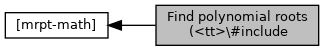MRPT  1.9.9
Find polynomial roots (`#include

## Detailed Description

Collaboration diagram for Find polynomial roots (`#include:## Functions

int mrpt::math::solve_poly3 (double *x, double a, double b, double c) noexcept
Solves cubic equation `x^3 + a*x^2 + b*x + c = 0`. More...

int mrpt::math::solve_poly4 (double *x, double a, double b, double c, double d) noexcept
Solves quartic equation `x^4 + a*x^3 + b*x^2 + c*x + d = 0` by Dekart-Euler method. More...

int mrpt::math::solve_poly5 (double *x, double a, double b, double c, double d, double e) noexcept
Solves equation `x^5 + a*x^4 + b*x^3 + c*x^2 + d*x + e = 0`. More...

int mrpt::math::solve_poly4Bi (double *x, double b, double d) noexcept
Solve equation x^4 + b*x^2 + d = 0. More...

int mrpt::math::solve_poly4De (double *x, double b, double c, double d) noexcept
Solve equation x^4 + b*x^2 + c*x + d = 0. More...

int mrpt::math::solve_poly2 (double a, double b, double c, double &r1, double &r2) noexcept
Solves equation `a*x^2 + b*x + c = 0`. More...

## ◆ solve_poly2()

 int mrpt::math::solve_poly2 ( double a, double b, double c, double & r1, double & r2 )
noexcept

Solves equation `a*x^2 + b*x + c = 0`.

Returns the number of real roots: either 0 or 2; or 1 if a=0 (in this case the root is in r1). r1, r2 are the roots. (r1<=r2)

Note
Based on `poly34.h`, by Khashin S.I. http://math.ivanovo.ac.ru/dalgebra/Khashin/index.html - khash2 (at) gmail.com

Definition at line 401 of file poly_roots.cpp.

References eps, and SWAP.

## ◆ solve_poly3()

 int mrpt::math::solve_poly3 ( double * x, double a, double b, double c )
noexcept

Solves cubic equation `x^3 + a*x^2 + b*x + c = 0`.

Returns the number of real roots `N`<=3. The roots are returned in the first entries of `x`, i.e. `x` if N=1, `x` and `x` if N=2, etc.

Parameters
 x array of size 3
Note
Based on `poly34.h`, by Khashin S.I. http://math.ivanovo.ac.ru/dalgebra/Khashin/index.html - khash2 (at) gmail.com

Definition at line 29 of file poly_roots.cpp.

References mrpt::obs::gnss::a2, eps, and TwoPi.

Referenced by SolveP4De(), TEST(), and mrpt::nav::CPTG_Holo_Blend::updateTPObstacleSingle().

## ◆ solve_poly4()

 int mrpt::math::solve_poly4 ( double * x, double a, double b, double c, double d )
noexcept

Solves quartic equation `x^4 + a*x^3 + b*x^2 + c*x + d = 0` by Dekart-Euler method.

Returns the number of real roots `N`<=4:

• return 4: 4 real roots x, x, x, x, possible multiple roots
• return 2: 2 real roots x, x and complex x+-i*x,
• return 0: two pair of complex roots: x+-i*x, x+-i*x,

The roots are returned in the first entries of `x`, i.e. `x` if N=1, `x` and `x` if N=2, etc.

Parameters
 x array of size 4
Note
Based on `poly34.h`, by Khashin S.I. http://math.ivanovo.ac.ru/dalgebra/Khashin/index.html - khash2 (at) gmail.com

Definition at line 254 of file poly_roots.cpp.

References mrpt::obs::gnss::b1, N4Step(), and SolveP4De().

## ◆ solve_poly4Bi()

 int mrpt::math::solve_poly4Bi ( double * x, double b, double d )
noexcept

Solve equation x^4 + b*x^2 + d = 0.

## ◆ solve_poly4De()

 int mrpt::math::solve_poly4De ( double * x, double b, double c, double d )
noexcept

Solve equation x^4 + b*x^2 + c*x + d = 0.

## ◆ solve_poly5()

 int mrpt::math::solve_poly5 ( double * x, double a, double b, double c, double d, double e )
noexcept

Solves equation `x^5 + a*x^4 + b*x^3 + c*x^2 + d*x + e = 0`.

Returns the number of real roots `N`<=5. The roots are returned in the first entries of `x`, i.e. `x` if N=1, `x` and `x` if N=2, etc.

Parameters
 x array of size 5
Note
Based on `poly34.h`, by Khashin S.I. http://math.ivanovo.ac.ru/dalgebra/Khashin/index.html - khash2 (at) gmail.com

Definition at line 388 of file poly_roots.cpp.

 Page generated by Doxygen 1.8.14 for MRPT 1.9.9 Git: 7d5e6d718 Fri Aug 24 01:51:28 2018 +0200 at lun nov 2 08:35:50 CET 2020